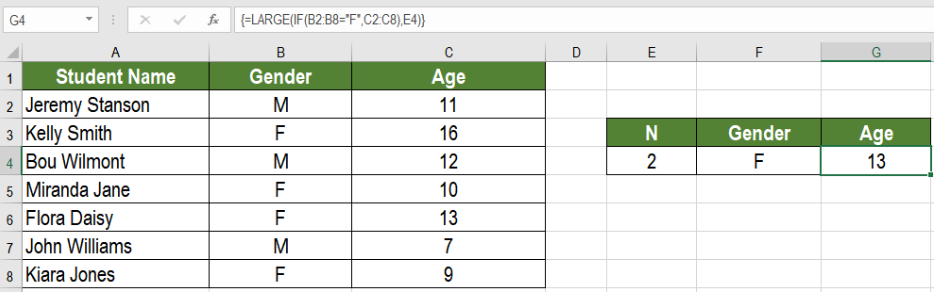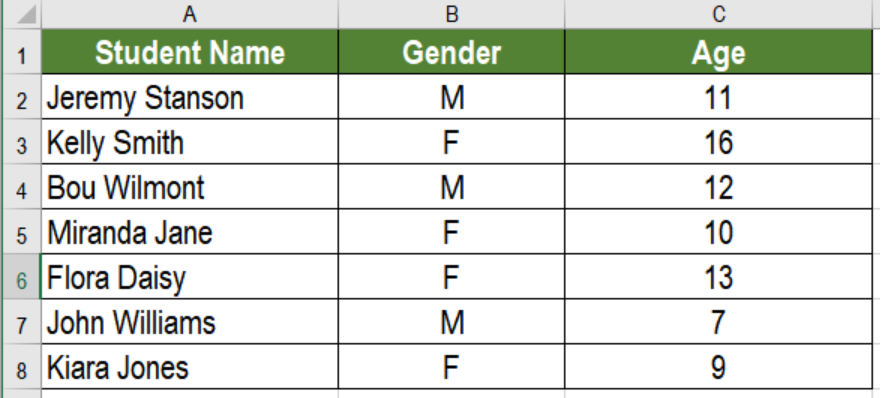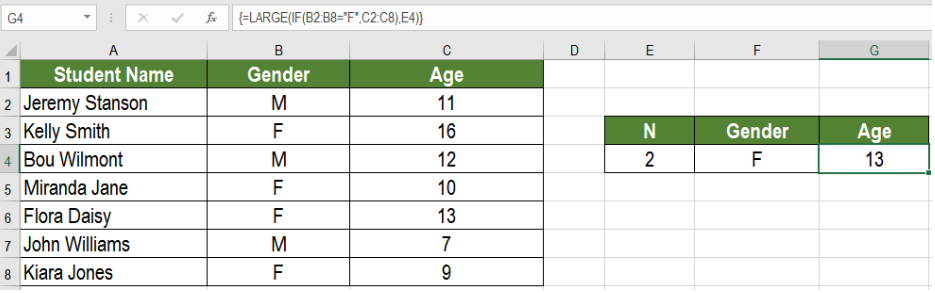Get instant live expert help with Excel or Google Sheets“My Excelchat expert helped me in less than 20 minutes, saving me what would have been 5 hours of work!”

#### Post your problem and you’ll get Expert help in seconds.

Your message must be at least 40 characters
Our professional Expert are available now. Your privacy is guaranteed.

# nth largest value with criteria

Excel offers some cool features to work with numbers. One of these is to find the nth largest value with criteria. Say, you have some numbers. With the help of Excel you can find the 2nd or 3rd largest number among them that satisfies a criteria. You can do it using the IF and LARGE functions. In this tutorial, we will learn how to find the nth largest value with criteria.Figure 1. Example of How to Find the nth Largest Value with Criteria

## Generic Formula

`=LARGE(IF(range=criteria,values),n)`

The main purpose of this formula is to find out the nth largest value. It is the value among the database which meets a specified criteria.

## Process

This formula uses the functions LARGE and IF. Firstly, You need to provide the range and an integer in place of the nth value.  This will fetch the nth value from the range. In order to satisfy the criteria, you need to apply the IF formula. Otherwise the LARGE function will be applied for every data and it will not meet the criteria. Hence, the use of the IF function providing a logical test to fulfil the criteria.

## Setting Up Data

The following example contains a student information database. Column A, B and C has the  name of the students, gender and age.Figure 2 .The Sample Data

To find the second oldest student, we need to:

• Go to cell G4.
• Assign the formula `=LARGE(IF(B2:B8="F",C2:C8),E4)` to G4.
• Press CTRL + SHIFT + ENTER to apply the formula to G4.Figure 3. Applying the Formula to the Data

Here, the criteria is the student needs to be female. Cell G4 has the age of the 2nd oldest female student named Flora Daisy, which is 13.

Excel has made working with numbers very simple. For example, we can find the nth largest value that satisfies a condition. The IF and LARGE functions help us to achieve this which we learnt in this lesson.

Most of the time, the problem you will need to solve will be more complex than a simple application of a formula or function. If you want to save hours of research and frustration, try our live Excelchat service! Our Excel Experts are available 24/7 to answer any Excel question you may have. We guarantee a connection within 30 seconds and a customized solution within 20 minutes.

### Did this post not answer your question? Get a solution from connecting with the expert.Another blog reader asked this question today on Excelchat:
Solution exampleshello i need help creating a formula, if a value does not exsits put 0, if a value exist do nothing
Solved by M. F. in 9 minsCompute the weighted average of students score from a spreadsheet using different weight for each category of marks
Solved by G. E. in 20 minshi there, im making a spread sheet to track progress in a game and would like to create a drop log sheet, i was hoping to use the if function to detect weather a item is logged on a sheet then send the sheet number to a list if so
Solved by K. F. in 60 minsI have a schedule I'm doing with formulas to add workdays (across rows) to a start date column. I would like to write the equation like this: =ifError((workday(b2,1) = cell above, [value of cell above + 1] Help me form this correctly to get the (value of target cell) = (1 more than the value from the cell above) IF the target cell value started equal to the cell above.
Solved by M. J. in 20 minsI need a formula that will subtract the sum of one cell if the sum of another cell is greater than zero, and have it applied to cells across a document that is 30,000 entries and only in certain spots
Solved by F. J. in 20 mins## Subscribe to Excelchat.coAnother blog reader asked this question today on Excelchat: